Next: Derivation of Kruppa's Equations Up: Self Calibration Previous: Self Calibration

## The Absolute Conic and the Camera Parameters

Consider two camera projections P and P' corresponding to the same camera (internal parameters) but different poses. We saw in exercise 4.3 that the image of the absolute conic (IAC) is independent of the camera pose. In fact, the IAC is directly related to the internal parameter matrix K of the camera defined in equation (1.2).

Given that the absolute conic is in any case invariant under rotations and translations, we can choose coordinates so that the first projection matrix reduces to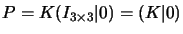(c.f. equation 1.1). Hence, for a point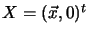on the plane at infinity we have projection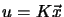or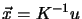.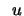is on the image of the absolute conic exactly when X is on the conic itself, i.e. when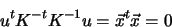So the image of the absolute conic is given by the matrix K-tK-1. If this matrix can be found, by Choleski factorization we can extract K-1 and thence find the internal parameter matrix K. In fact, as we will see, it is easier to work from the outset with the inverse KtK of the IAC matrix, called the dual image of the absolute conic (DIAC).Next: Derivation of Kruppa's Equations Up: Self Calibration Previous: Self Calibration
Bill Triggs
1998-11-13